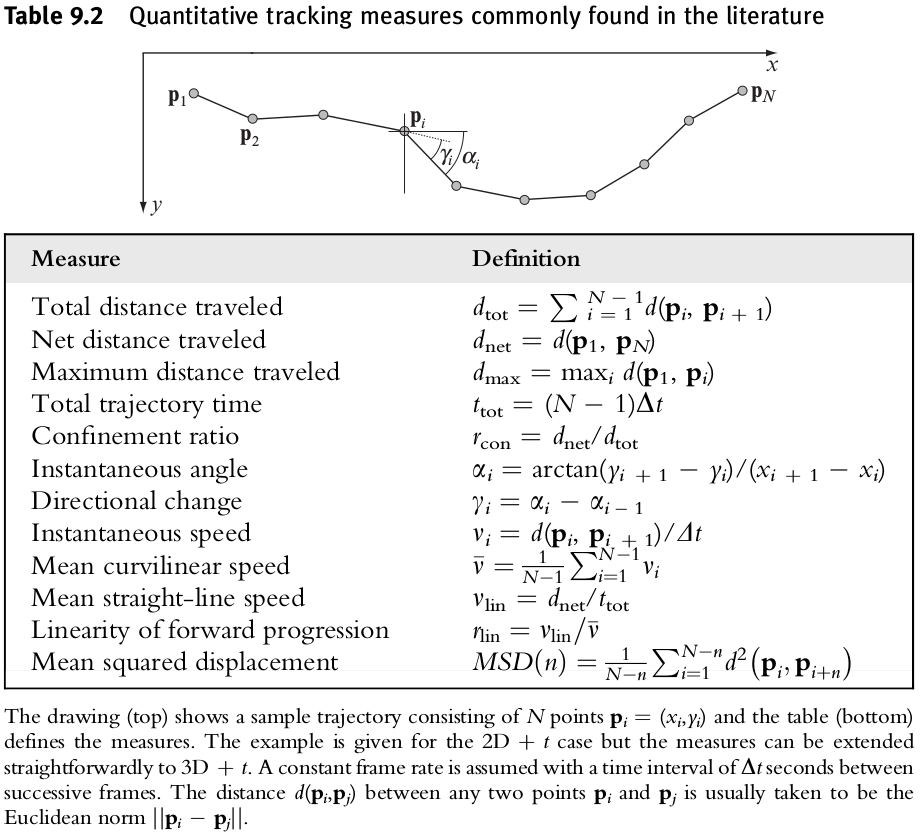# Methods for Cell Tracking

## Abstract

A recurring task: the tracking of large numbers of cells or particles and the analysis of their (morpho)dynamic behavior.

## Introduction

• spatial (anatomic) and temporal (dynamic) properties
• the development of a vast spectrum of fluorescent proteins and nanocrystals and groundbreaking advances in optical microscopy technology made live imaging of dynamic processes at the cellular and molecular levels.

## Tracking Approaches

Generally, two sides to the tracking problem,

• the segmentation step: the recognition of relevant objects and their separation from the background in every frame
• the linking step: the association of segmented objects from frame to frame and making connections

### Cell tracking approaches

segmentation:

• easy way: thresholding
• sophisticated segmentation approaches: template matching …

• simplest: link every segmented cell in any given frame to the nearest cell in the next frame, where “nearest” may refer not only to spatial distance but also to difference in intensity, volume, orientation, and other features.
• implicit way along segmentation: template matching …

### Particle tracking approaches

Individual proteins or other (macro) molecular complexes within cells (collectively referred to as particles) are hardly (if at all) visible in bright field or phase-contrast microscopy and require fluorescent labeling and imaging.

## Tracking tools

• CellProfiler: https://cellprofiler.org/

## Tracking Measures

### Motility measures### Diffusivity measures

• pure random walk (such as Brownian motion of particles): $MSD(t) = cDt$, with $c=4$ in 2D and $c=6$ in 3D, and where $D$ denotes the so-called diffusion coefficient.
• motion impeded by obstacles: $MSD(t)=cDt^\alpha$ with $\alpha < 1$.
• motion confined to some region: $MSD(t)=R[1-a_1\exp(-a_2cDt/R)]$.
• directed motion (flow) in addition to diffusion: $MSD(t)=cDt+(vt)^2$.

### Morphology measures

• measures of size and orientation: perimeter (surface area), area (volume), and the major and minor axes.
• measures of (size and orientation invariant) complexity: circularity (sphericity), eccentricity (ellipticity), and convexity or concavity
• more sophisticated analysis of morphology: decompose the shape in terms of Zernike polynomials or based on Fourier analysis, independent component analysis, or principal component analysis

## Tips and Tricks

Imaging:

• dimensionality
• image quality: SNR
• frame rate

Tracking:

• preprocessing
• tool selection
• verification

Analysis:

• diffusivity
• velocity
• aggregation: object based (a histogram of the per-object mean speeds will reveal objects consisting of two subpopulations) or frame based (detection of different modes of motion).

Published in categories Note